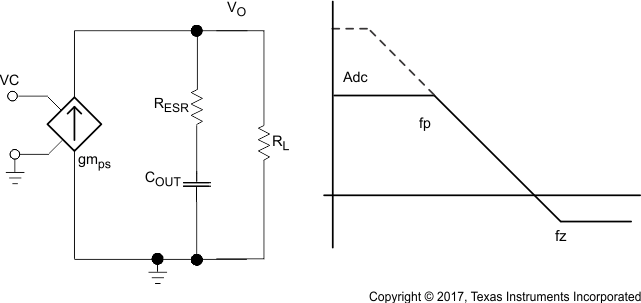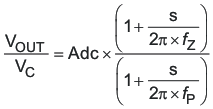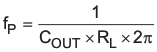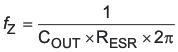JAJSBY0G August   2012  – June 2018

PRODUCTION DATA.

1. 特長
2. アプリケーション
3. 概要
1.     Device Images
4. 改訂履歴
5. Pin Configuration and Functions
6. Specifications
7. Detailed Description
1. 7.1 Overview
2. 7.2 Functional Block Diagram
3. 7.3 Feature Description
4. 7.4 Device Functional Modes
8. Application and Implementation
1. 8.1 Application Information
2. 8.2 Typical Application
1. 8.2.1 Design Requirements
2. 8.2.2 Detailed Design Procedure
3. 8.2.3 Application Curves
9. Power Supply Recommendations
10. 10Layout
1. 10.1 Layout Guidelines
2. 10.2 Layout Example
11. 11デバイスおよびドキュメントのサポート
12. 12メカニカル、パッケージ、および注文情報

• DDA|8
• DDA|8

7.3.15 Simple Small Signal Model for Peak Current Mode Control

Figure 29 describes a simple small signal model that can be used to design the frequency compensation. The TPS54360 power stage can be approximated by a voltage-controlled current source (duty cycle modulator) supplying current to the output capacitor and load resistor. The control to output transfer function is shown in Equation 11 and consists of a dc gain, one dominant pole, and one ESR zero. The quotient of the change in switch current and the change in COMP terminal voltage (node c in Figure 28) is the power stage transconductance, gmPS. The gmPS for the TPS54360 is 12 A/V. The low-frequency gain of the power stage is the product of the transconductance and the load resistance as shown in Equation 12.

As the load current increases and decreases, the low-frequency gain decreases and increases, respectively. This variation with the load may seem problematic at first glance, but fortunately the dominant pole moves with the load current (see Equation 13). The combined effect is highlighted by the dashed line in the right half of Figure 29. As the load current decreases, the gain increases and the pole frequency lowers, keeping the 0-dB crossover frequency the same with varying load conditions. The type of output capacitor chosen determines whether the ESR zero has a profound effect on the frequency compensation design. Using high ESR aluminum electrolytic capacitors may reduce the number frequency compensation components needed to stabilize the overall loop because the phase margin is increased by the ESR zero of the output capacitor (see Equation 14).Figure 29. Simple Small Signal Model and Frequency Response for Peak Current Mode Control
Equation 11.Equation 12.Equation 13.Equation 14.